# Colored blocks

Tucker and his classmates placed colored blocks on a scale during a science lab. The brown block weighed 8.94 pounds, and the red block weighed 1.87 pounds. How much more did the brown block weigh than the red block?

d =  7.07 lb

### Step-by-step explanation: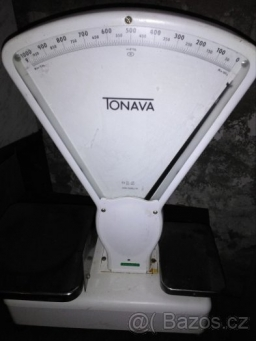Did you find an error or inaccuracy? Feel free to write us. Thank you!Tips to related online calculators
Need help to calculate sum, simplify or multiply fractions? Try our fraction calculator.

## Related math problems and questions:

• Cement blocks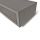Each cement block weighs 7.9 kilograms. How much do 6 blocks weigh in total?
• Pounds3 pounds subtract 1/3 of a pound.
• Colored paperCan a 4 cm, 3 cm and 2 cm block be glued with a colored quarter of paper measuring 7 cm and 8 cm?
• Comparing and sortingArrange in descending order this fractions: 2/7, 7/10 & 1/2
• Bent scaleMonica weighed 52 kg. Sara 54 kg. Together they weighed 111 kg. They noticed that the weight on the scale was bent. How much did they really weigh?
• Dividing walnuts into crates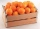There are 8 and 2 over 3 pounds of walnuts in a container, which will be divided equally into containers that hold 1 and 1 over 5 pounds. This would fill n and 4 over 18 containers. What is n?
• Brick weightHalf the weight of a brick plus 20 pounds is equal to 1/3 the weight of the brick plus 30 pounds. How much does the brick weigh?
• The dough 2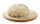A baker made 8 pounds of dough. He used 5/9 of the dough to make sandwich rolls. How much of the dough is left over?
• Wooden blockA cuboid-shaped wooden block has 6 cm length, 4 cm breadth, and 1 cm height. Two faces measuring 4 cm x 1 cm are colored in black. Two faces measuring 6 cm x 1 cm are colored in red. Two faces measuring 6 cm x 4 cm are colored in green. The block is divid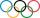Pupils doing research which a winter sport do their classmates most popular. They found that 2/5 of classmates would most like to play hockey, skate prefer 2/9 pupils, 3/10 students prefer skiing and 1/15 classmates don't like any winter sport. What propo
• A supermarket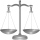A supermarket has 3 7/8 pounds of tangerines. Kelsey buys 1/2 of all the tangerines in the supermarket. How many pounds of tangerines did Kelsey buy?
• Split between 4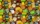There are 12 1/4 pounds of candy canes to split between four classes evenly. How many pounds of the candy canes will each class get?
• CompareCompare fractions (34)/(3) and (12)/(4). Which fraction of the lower?
• Weigh in totalI put 3/5 kg of grapes into a box which is 1/4kg in weight. How many kilograms do the grapes and the box weigh in total?
• Flowers 4A flower seller has 4.05 kg of orange flowers and 6.50 kg of red flowers. He made flower baskets with 2.11 kg of both red and orange flowers in each. How many baskets did he make?
• FractionFind for what x fraction (-4x -6)/(x) equals:
• LarryLarry received 1/3 pound of candy from his grandmother and 2/5 pound of candy from his best friend. Larry's sister ate 1/2 pound of Larry's candy. How many pounds of candy does Larry have left?